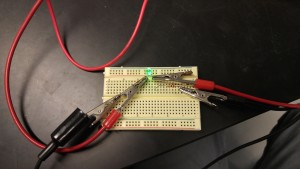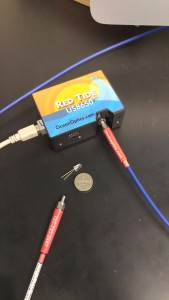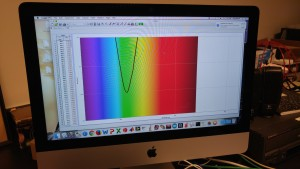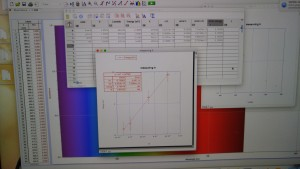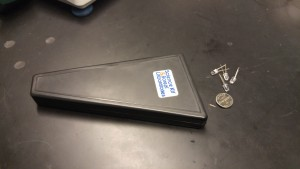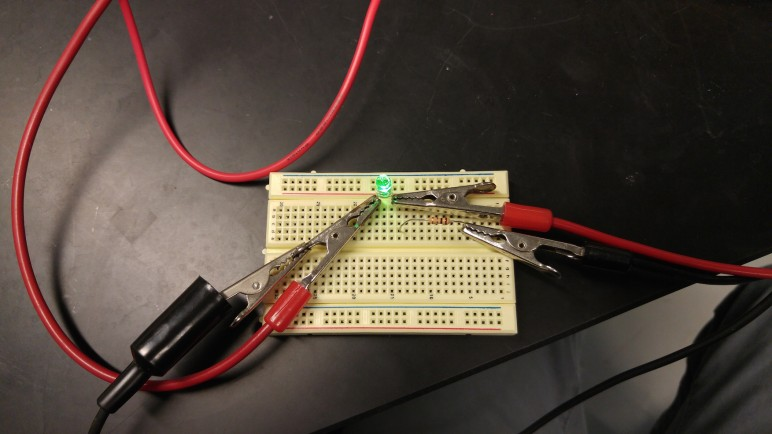# Measuring h with LED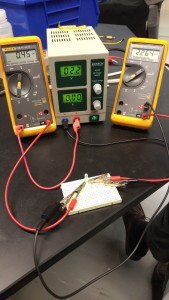How to measure Planck’s constant using an LED

• Measure LED threshold voltage using voltmeter and ammeter.
• LED converts electron energy to photons. Energy lost by electron = energy gained by photon = (electron charge) times (voltage).
• So, if threshold voltage is 2.2 volts, energy of emitted photon must be 2.2 eV.
• Use  E=hc/lambda, and measure lambda using Red Tide spectrometer with Logger Pro (see below pic), to determine h. Take data using LEDs of 4 different colors and fit data to line with slope h.
• Hand held spectrometer can be used to view LED spectrum.